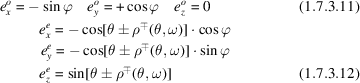International
Tables for
Crystallography
Volume D
Physical properties of crystals
Edited by A. Authier

International Tables for Crystallography (2013). Vol. D, ch. 1.7, pp. 188-189

## Section 1.7.3.1.3. Uniaxial class

B. Boulangera* and J. Zyssb

aInstitut Néel CNRS Université Joseph Fourier, 25 rue des Martyrs, BP 166, 38042 Grenoble Cedex 9, France, and bLaboratoire de Photonique Quantique et Moléculaire, Ecole Normale Supérieure de Cachan, 61 Avenue du Président Wilson, 94235 Cachan, France
Correspondence e-mail:  benoit.boulanger@grenoble.cnrs.fr

#### 1.7.3.1.3. Uniaxial class

| top | pdf |

The uniaxial class is characterized by the equality of two principal indices, called ordinary indices (); the other index is called the extraordinary index (). Then, according to (1.7.3.6), the index surface has one umbilicus along the z axis,, called the optic axis, which is along the fold rotation axis of greatest order of the crystal. The two other principal axes are related to the symmetry elements of the orientation class according to the standard conventions (Nye, 1957). The ordinary sheet is spherical i.e., so an ordinary wave has no walk-off for any direction of propagation in a uniaxial crystal; the extraordinary sheet is ellipsoidal i.e.. The sign of the uniaxial class is defined by the sign of the birefringence. Thus, according to these definitions, () corresponds to () for the positive class () and to () for the negative class (), as shown in Fig. 1.7.3.2.Figure 1.7.3.2 | top | pdf |Index surfaces of the negative and positive uniaxial classes.are the ordinary (o) and extraordinary (e) electric field vectors relative to the external (+) or internal (−) sheets. OA is the optic axis.

The ordinary electric field vector is orthogonal to the optic axis (), and also to the extraordinary electric field vector, leading toThis relation is satisfied when ωi and ωj are equal or different and for any direction of propagation ().

According to these results, the coplanarity of the field vectors imposes the condition that the double-refraction angle of the extraordinary wave is in a plane containing the optic axis. Thus, the components of the ordinary and extraordinary unit electric field vectors eo and ee at the circular frequency ω arewithfor the positive class andfor the negative class.is given byNote that the extraordinary walk-off angle is nil for a propagation along the optic axis () and everywhere in the xy plane ().

### References

Nye, J. F. (1957). Physical properties of crystals. Oxford: Clarendon Press.